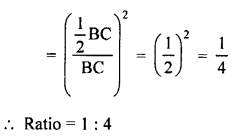## RS Aggarwal Class 10 Solutions Chapter 4 Triangles Ex 4C

These Solutions are part of RS Aggarwal Solutions Class 10. Here we have given RS Aggarwal Solutions Class 10 Chapter 4 Triangles Ex 4C.

Other Exercises

Question 1.
Solution:
Given : Area of ∆ABC = 64 cm²
and area of ∆DEF =121 cm²
EF = 15.4 cm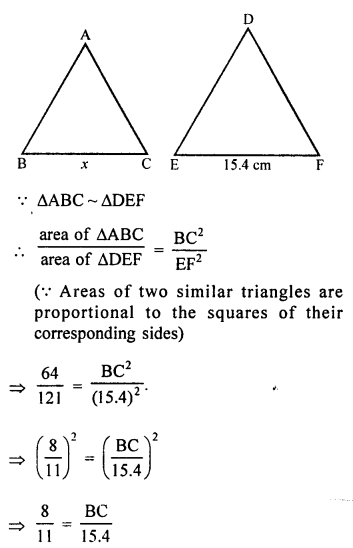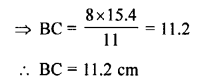Question 2.
Solution: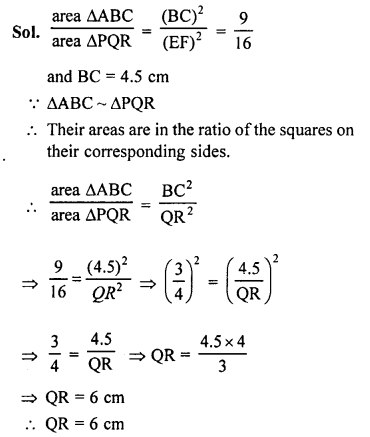Question 3.
Solution:
∆ABC ~ ∆PQR
ar (∆ABC) = 4ar (∆PQR),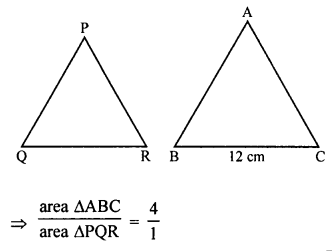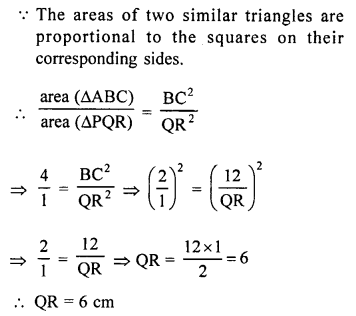Question 4.
Solution:
Areas of two similar triangles are 169 cm² and 121 cm²
Longest side of largest triangle = 26 cm
Let longest side of smallest triangle = x
∆s are similar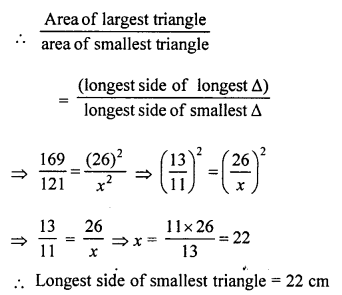Question 5.
Solution:
Area of ∆ABC = 100 cm²
and area of ∆DEF = 49 cm²Question 6.
Solution:
Given : Corresponding altitudes of two similar triangles are 6 cm and 9 cm
We know that the areas of two similar triangles are in the ratio of the squares of their corresponding altitudes.
Ratio in the areas of two similar triangles = (6)² : (9)² = 36 : 81 = 4 : 9 (Dividing by 9)

Question 7.
Solution:
The areas of two similar triangles are 81 cm² and 49 cm²
Altitude of the first triangle = 6.3 cm
Let altitude of second triangle = x cm
The areas of two similar triangles are in the ratio of the squares on their corresponding altitude,
Let area of ∆ABC = 81 cm²
and area of ∆DEF = 49cm²
Altitude AL = 6 – 3 cm
Let altitude DM = x cm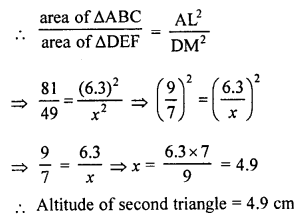Question 8.
Solution:
Areas of two similar triangles are 100 cm² and 64 cm²
Let area of ∆ABC = 100 cm²
and area of ∆DEF = 64 cm²
Median DM of ∆DEF = 5.6 cm
Let median AL of ∆ABC = x
The areas of two similar triangles is proportional to the squares of their corresponding median.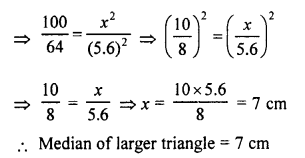Question 9.
Solution:
Given : In ∆ABC, PQ is a line which meets AB in P and AC in Q.
AP = 1 cm, PB = 3 cm, AQ = 1.5 cm QC = 4.5 cm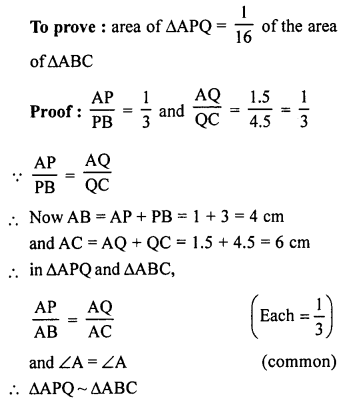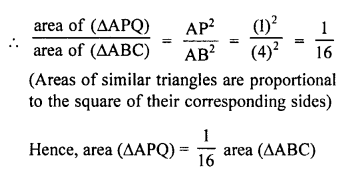Question 10.
Solution:
In ∆ABC,
DE || BC
DE = 3 cm, BC = 6 cm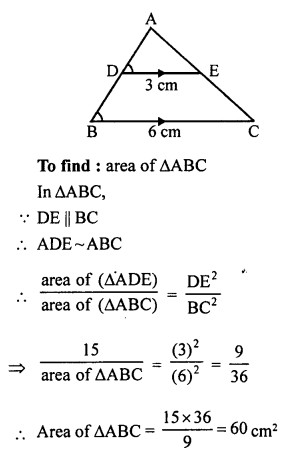Question 11.
Solution:
Given : In right ∆ABC, ∠A = 90°
BC = 13 cm, AC = 5 cm
To find : Ratio in area of ∆ABC and ∆ADC
∠C = ∠C (common)Question 12.
Solution:
In the given figure ∆ABC,
DE || BC and DE : BC = 3 : 5.
DE || BC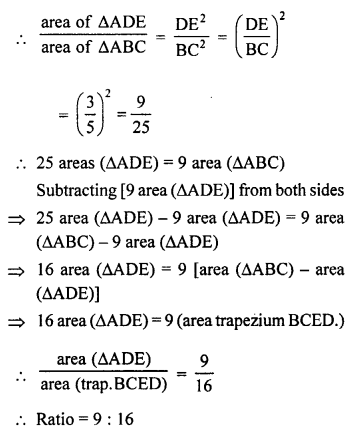DE || BC and DE = $$\frac { 1 }{ 2 }$$ BC